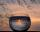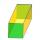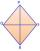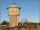Two balls

Two balls, one 8cm in radius and the other 6cm in radius, are placed in a cylindrical plastic container 10cm in radius. Find the volume of water necessary to cover them.

Result

V =  5322.626 cm3

Solution:Leave us a comment of example and its solution (i.e. if it is still somewhat unclear...):Be the first to comment!To solve this verbal math problem are needed these knowledge from mathematics:

Tip: Our volume units converter will help you with converion of volume units. Pythagorean theorem is the base for the right triangle calculator.

Next similar examples:

1. Cube in sphereThe sphere is inscribed cube with edge 8 cm. Find the radius of the sphere.
2. Truncated cone 5The height of a cone 7 cm and the length of side is 10 cm and the lower radius is 3cm. What could the possible answer for the upper radius of truncated cone?
3. Water wellDrilled well has a depth 20 meters and 0.1 meters radius. How many liters of water can fit into the well?
4. Cylinder - A&VThe cylinder has a volume 1287. The base has a radius 10. What is the area of surface of the cylinder?
5. RainHow many mm of water rained the roof space 75 m2 if the empty barrel with a radius of 8 dm and height 1.2 m filled to 75% its capacity? :-)
6. Diagonals in diamons/rhombusRhombus ABCD has side length AB = 4 cm and a length of one diagonal of 6.4 cm. Calculate the length of the other diagonal.
7. SatinSanusha buys a piece of satin 2.4 m wide. The diagonal length of the fabric is 4m. What is the length of the piece of satin?
8. TetrahedronCalculate height and volume of a regular tetrahedron whose edge has a length 18 cm.
9. PrismThe lenght, width and height of a right prism are 6, 17 and 10 respectively. What is the lenght of the longest segment whose endpoints are vertices of the prism?
10. 4s pyramidRegular tetrahedral pyramid has a base edge a=17 and collaterally edge length b=32. What is its height?
11. DiamondCalculate the length of the two diagonals of the diamond if: a = 13 cm v = 12 cm
12. DistanceWha is the distance between the origin and the point (18; 22)?
13. Water reservoirThe reservoir shape of a cylinder having a diameter 8 m and a height 2.8 m. The device indicates that the reservoir contains 800 hectoliters of water. Calculate how much % of volume is used.
14. Theorem proveWe want to prove the sentence: If the natural number n is divisible by six, then n is divisible by three. From what assumption we started?
15. GasholderThe gasholder has spherical shape with a diameter 20 m. How many m3 can hold in?
16. Total displacementCalculate total displacement of the 4-cylinder engine with the diameter of the piston bore B = 6.6 cm and stroke S=2.4 cm of the piston. Help: the crankshaft makes one revolution while the piston moves from the top of the cylinder to the bottom and back.
17. Garden pondConcrete garden pond has bottom shape of a semicircle with a diameter 1.7 m and is 79 cm deep. Daddy wants make it surface. How many liters of water is in pond if watel level is 28 cm?